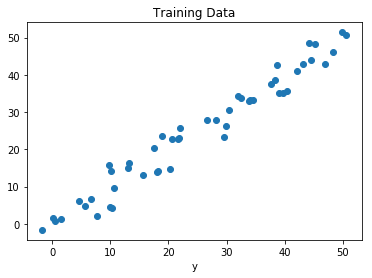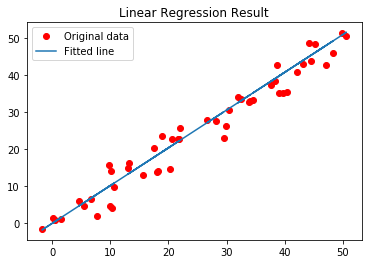# Linear Regression Using Tensorflow

## Brief Summary of Linear Regression

Linear Regression is a very common statistical method that allows us to learn a function or relationship from a given set of continuous data. For example, we are given some data points of `x` and corresponding `y` and we need to learn the relationship between them that is called a hypothesis.

In case of Linear regression, the hypothesis is a straight line, i.e,Where `w` is a vector called Weights and `b` is a scalar called Bias. The Weights and Bias are called the parameters of the model.

All we need to do is estimate the value of w and b from the given set of data such that the resultant hypothesis produces the least cost `J` which is defined by the following cost functionwhere `m` is the number of data points in the given dataset. This cost function is also called Mean Squared Error.

For finding the optimized value of the parameters for which `J` is minimum, we will be using a commonly used optimizer algorithm called Gradient Descent. Following is the pseudo-code for Gradient Descent:

` `

```Repeat untill Convergence {
w = w - α * δJ/δw
b = b - α * δJ/δb
}
```

where `α` is a hyperparameter called the Learning Rate.

## Tensorflow

Tensorflow is an open-source computation library made by Google. It is a popular choice for creating applications that require high-end numerical computations and/or need to utilize Graphics Processing Units for computation purposes. These are the main reasons due to which Tensorflow is one of the most popular choices for Machine Learning applications, especially Deep Learning. It also has APIs like Estimator which provide a high level of abstraction while building Machine Learning Applications. In this article, we will not be using any high-level APIs, rather we will be building the Linear Regression model using low-level Tensorflow in the Lazy Execution Mode during which Tensorflow creates a Directed Acyclic Graph or DAG which keeps track of all the computations, and then executes all the computations done inside a Tensorflow Session.

## Implementation

We will start by importing the necessary libraries. We will use Numpy along with Tensorflow for computations and Matplotlib for plotting.

 `import` `numpy as np ` `import` `tensorflow as tf ` `import` `matplotlib.pyplot as plt `

In order to make the random numbers predictable, we will define fixed seeds for both Numpy and Tensorflow.

 `np.random.seed(``101``) ` `tf.set_random_seed(``101``) `

Now, let us generate some random data for training the Linear Regression Model.

 `# Genrating random linear data ` `# There will be 50 data points ranging from 0 to 50 ` `x ``=` `np.linspace(``0``, ``50``, ``50``) ` `y ``=` `np.linspace(``0``, ``50``, ``50``) ` ` ` `# Adding noise to the random linear data ` `x ``+``=` `np.random.uniform(``-``4``, ``4``, ``50``) ` `y ``+``=` `np.random.uniform(``-``4``, ``4``, ``50``) ` ` ` `n ``=` `len``(x) ``# Number of data points `

Let us visualize the training data.

 `# Plot of Training Data ` `plt.scatter(x, y) ` `plt.xlabel(``'x'``) ` `plt.xlabel(``'y'``) ` `plt.title(``"Training Data"``) ` `plt.show() `

Output:

````

Now we will start creating our model by defining the placeholders `X` and `Y`, so that we can feed our training examples `X` and `Y` into the optimizer during the training process.

 `X ``=` `tf.placeholder(``"float"``) ` `Y ``=` `tf.placeholder(``"float"``) `

Now we will declare two trainable Tensorflow Variables for the Weights and Bias and initializing them randomly using `np.random.randn()`.

 `W ``=` `tf.Variable(np.random.randn(), name ``=` `"W"``) ` `b ``=` `tf.Variable(np.random.randn(), name ``=` `"b"``) `

Now we will define the hyperparameters of the model, the Learning Rate and the number of Epochs.

 `learning_rate ``=` `0.01` `training_epochs ``=` `1000`

Now, we will be building the Hypothesis, the Cost Function, and the Optimizer. We won’t be implementing the Gradient Descent Optimizer manually since it is built inside Tensorflow. After that, we will be initializing the Variables.

 `# Hypothesis ` `y_pred ``=` `tf.add(tf.multiply(X, W), b) ` ` ` `# Mean Squared Error Cost Function ` `cost ``=` `tf.reduce_sum(tf.``pow``(y_pred``-``Y, ``2``)) ``/` `(``2` `*` `n) ` ` ` `# Gradient Descent Optimizer ` `optimizer ``=` `tf.train.GradientDescentOptimizer(learning_rate).minimize(cost) ` ` ` `# Global Variables Initializer ` `init ``=` `tf.global_variables_initializer() `

Now we will begin the training process inside a Tensorflow Session.

 `# Starting the Tensorflow Session ` `with tf.Session() as sess: ` `     ` `    ``# Initializing the Variables ` `    ``sess.run(init) ` `     ` `    ``# Iterating through all the epochs ` `    ``for` `epoch ``in` `range``(training_epochs): ` `         ` `        ``# Feeding each data point into the optimizer using Feed Dictionary ` `        ``for` `(_x, _y) ``in` `zip``(x, y): ` `            ``sess.run(optimizer, feed_dict ``=` `{X : _x, Y : _y}) ` `         ` `        ``# Displaying the result after every 50 epochs ` `        ``if` `(epoch ``+` `1``) ``%` `50` `=``=` `0``: ` `            ``# Calculating the cost a every epoch ` `            ``c ``=` `sess.run(cost, feed_dict ``=` `{X : x, Y : y}) ` `            ``print``(``"Epoch"``, (epoch ``+` `1``), ``": cost ="``, c, ``"W ="``, sess.run(W), ``"b ="``, sess.run(b)) ` `     ` `    ``# Storing necessary values to be used outside the Session ` `    ``training_cost ``=` `sess.run(cost, feed_dict ``=``{X: x, Y: y}) ` `    ``weight ``=` `sess.run(W) ` `    ``bias ``=` `sess.run(b) `

Output:

``````Epoch: 50 cost = 5.8868036 W = 0.9951241 b = 1.2381054
Epoch: 100 cost = 5.7912707 W = 0.99812365 b = 1.0914398
Epoch: 150 cost = 5.7119675 W = 1.0008028 b = 0.96044314
Epoch: 200 cost = 5.6459413 W = 1.0031956 b = 0.8434396
Epoch: 250 cost = 5.590799 W = 1.0053328 b = 0.7389357
Epoch: 300 cost = 5.544608 W = 1.007242 b = 0.6455922
Epoch: 350 cost = 5.5057883 W = 1.008947 b = 0.56222
Epoch: 400 cost = 5.473066 W = 1.01047 b = 0.48775345
Epoch: 450 cost = 5.4453845 W = 1.0118302 b = 0.42124167
Epoch: 500 cost = 5.421903 W = 1.0130452 b = 0.36183488
Epoch: 550 cost = 5.4019217 W = 1.0141305 b = 0.30877414
Epoch: 600 cost = 5.3848577 W = 1.0150996 b = 0.26138115
Epoch: 650 cost = 5.370246 W = 1.0159653 b = 0.21905091
Epoch: 700 cost = 5.3576994 W = 1.0167387 b = 0.18124212
Epoch: 750 cost = 5.3468933 W = 1.0174294 b = 0.14747244
Epoch: 800 cost = 5.3375573 W = 1.0180461 b = 0.11730931
Epoch: 850 cost = 5.3294764 W = 1.0185971 b = 0.090368524
Epoch: 900 cost = 5.322459 W = 1.0190892 b = 0.0663058
Epoch: 950 cost = 5.3163586 W = 1.0195289 b = 0.044813324
Epoch: 1000 cost = 5.3110332 W = 1.0199214 b = 0.02561663``````

Now let us look at the result.

 `# Calculating the predictions ` `predictions ``=` `weight ``*` `x ``+` `bias ` `print``(``"Training cost ="``, training_cost, ``"Weight ="``, weight, ``"bias ="``, bias, ``'n'``) `

Output:

``Training cost = 5.3110332 Weight = 1.0199214 bias = 0.02561663``

Note that in this case both the Weight and bias are scalars. This is because, we have considered only one dependent variable in out training data. If we have m dependent variables in our training dataset, the Weight will be an m-dimensional vector while bias will be a scalar.

Finally, we will plot our result.

 `# Plotting the Results ` `plt.plot(x, y, ``'ro'``, label ``=``'Original data'``) ` `plt.plot(x, predictions, label ``=``'Fitted line'``) ` `plt.title(``'Linear Regression Result'``) ` `plt.legend() ` `plt.show() `

Output:

````

# Special 95% discount

## 2000+ Applied Machine Learning & Data Science Recipes

### Portfolio Projects for Aspiring Data Scientists: Tabular Text & Image Data Analytics as well as Time Series Forecasting in Python & R## Two Machine Learning Fields

There are two sides to machine learning:

• Practical Machine Learning:This is about querying databases, cleaning data, writing scripts to transform data and gluing algorithm and libraries together and writing custom code to squeeze reliable answers from data to satisfy difficult and ill defined questions. It’s the mess of reality.
• Theoretical Machine Learning: This is about math and abstraction and idealized scenarios and limits and beauty and informing what is possible. It is a whole lot neater and cleaner and removed from the mess of reality.

Data Science Resources: Data Science Recipes and Applied Machine Learning Recipes

Introduction to Applied Machine Learning & Data Science for Beginners, Business Analysts, Students, Researchers and Freelancers with Python & R Codes @ Western Australian Center for Applied Machine Learning & Data Science (WACAMLDS) !!!

Latest end-to-end Learn by Coding Recipes in Project-Based Learning:

Applied Statistics with R for Beginners and Business Professionals

Data Science and Machine Learning Projects in Python: Tabular Data Analytics

Data Science and Machine Learning Projects in R: Tabular Data Analytics

Python Machine Learning & Data Science Recipes: Learn by Coding

R Machine Learning & Data Science Recipes: Learn by Coding

Comparing Different Machine Learning Algorithms in Python for Classification (FREE)

`Disclaimer: The information and code presented within this recipe/tutorial is only for educational and coaching purposes for beginners and developers. Anyone can practice and apply the recipe/tutorial presented here, but the reader is taking full responsibility for his/her actions. The author (content curator) of this recipe (code / program) has made every effort to ensure the accuracy of the information was correct at time of publication. The author (content curator) does not assume and hereby disclaims any liability to any party for any loss, damage, or disruption caused by errors or omissions, whether such errors or omissions result from accident, negligence, or any other cause. The information presented here could also be found in public knowledge domains.  `